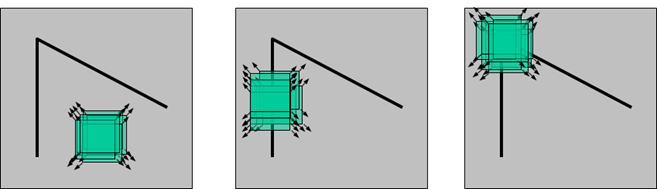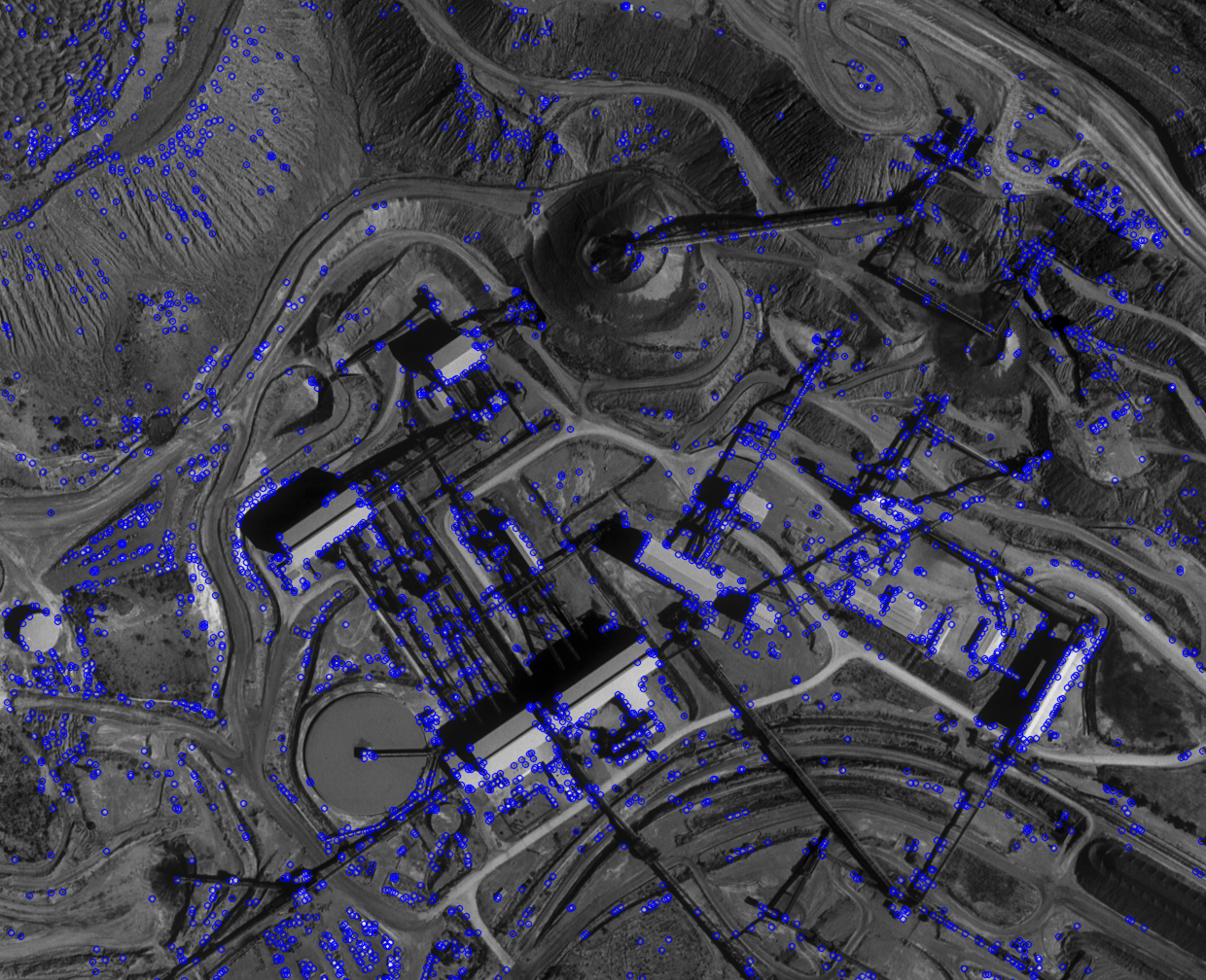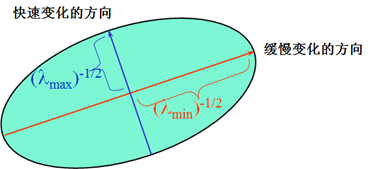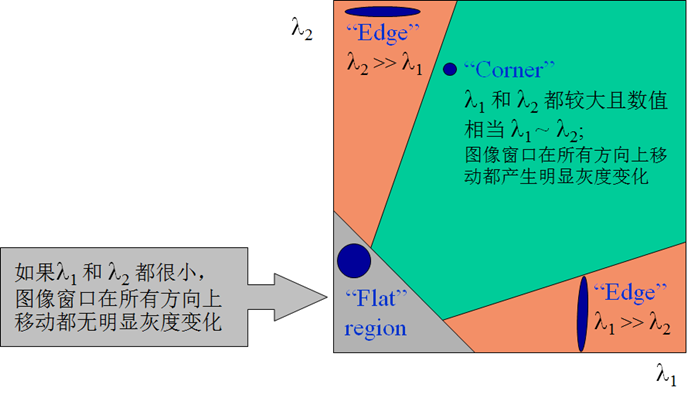# OpenCV——点特征提取算子：Moravec，Forstner 与 Harris

## Interest Operators: Moravec, Forstner & Harris

Thistledown    April 2, 2019

• 边缘（Edges
• 角点（Corners
• 兴趣区域 ROI（Regions of Interest## Moravec 算子

#### 基本原理

Moravec 于 1977 年提出利用灰度方差提取点特征的算子，其步骤为：

1. 计算各像元的兴趣值 $IV$（Interest Value）。 在以像素 $(c, r)$ 为中心的 $w \times w$ 的窗口中（如 $5 \times 5$ 的窗口），计算四个方向相邻像素灰度差的平方和：

2. 给定一经验阈值，将兴趣值大于该阈值的点（即兴趣值计算窗口的中心点）作为候选点。 阈值的选择应以候选点中包括所需要的特征点而又不含过多的非特征点为原则。

3. 选取候选点中的极值点作为特征点。 在一定大小的窗口内（可不同于兴趣值计算窗口，例如 $5 \times 5$ 像元，$7 \times 7$ 像元或 $9 \times 9$ 像元），将候选点中兴趣值不是最大者均去掉，仅留下一个兴趣值最大者，该像素即为一个特征点（有的文献中称此步骤为抑制局部非最大）。

#### 代码实现

1
2
3
4
5
6
7
8
9
10
11
12
13
14
15
16
17
18
19
20
21
22
23
24
25
26
27
28
29
30
31
32
33
34
35
36
37
38
39
40
41
42
43
44
45
46
47
48
49
50
51
52
53
54
55
56
57
58
59
60
61
62
63
64
65
66
67
68
69
/// Moravec 点特征提取算法
void Moravec(InputArray image, vector<KeyPoint>& keyPoints, int kSize, int threshold) {
Mat img;
cvtColor(image, img, COLOR_BGR2GRAY);

unsigned char* pImg = img.data;
int height = img.rows;
int width = img.cols;
int size = height * width;

double* candidatePoints = new double[size];
for (int i = 0; i < size; i++)
candidatePoints[i] = 0;

int k = kSize / 2;

/// I. 计算各像元的兴趣值
for (int i = k; i < height - k; i++) {
for (int j = k; j < width - k; j++) {
int V1, V2, V3, V4;
V1 = V2 = V3 = V4 = 0;

// 在以像素 (i, j) 为中心的 k * k 的影像窗口中
// 计算水平、45°、竖直、135°四个方向上相邻像素灰度差的平方和
for (int r = -k; r < k; r++) {
V1 += (pImg[(i + r) * width + j] - pImg[(i + r + 1) * width + j]) *
(pImg[(i + r) * width + j] - pImg[(i + r + 1) * width + j]);
V2 += (pImg[(i + r) * width + j + r] - pImg[(i + r + 1) * width + j + r + 1]) *
(pImg[(i + r) * width + j + r] - pImg[(i + r + 1) * width + j + r + 1]);
V3 += (pImg[i * width + j + r] - pImg[i * width + j + r + 1]) *
(pImg[i * width + j + r] - pImg[i * width + j + r + 1]);
V4 += (pImg[(i + r) * width + j - r] - pImg[(i + r + 1) * width + j - r - 1]) *
(pImg[(i + r) * width + j - r] - pImg[(i + r + 1) * width + j - r - 1]);
}

// 取其中的最小值作为该像素 (i, j) 的兴趣值
int value = min(min(V1, V2), min(V3, V4));

// II. 将兴趣值大于阈值的点作为候选点
if (value > threshold)
candidatePoints[i * width + j] = value;
}
}

/// III. 选取候选点中的极值点作为特征点（非极大值抑制）
int max_i, max_j, flag;
double max_val;
for (int i = k; i < height - k; i += kSize) {
for (int j = k; j < width - k; j += kSize) {
max_i = 0;
max_j = 0;
max_val = 0;
flag = 0;
for (int m = -k; m < k; m++) {
for (int n = -k; n < k; n++) {
double val = candidatePoints[(i + m) * width + j + n];
if (val > max_val) {
max_i = i + m;
max_j = j + n;
max_val = val;
flag = 1;
}
}
}
if (flag)
keyPoints.push_back(KeyPoint((float)max_j, (float)max_i, 2));
}
}
}


#### 提取结果## Forstner 算子

#### 基本原理

1. 计算各像素的 Robert 梯度。

2. 计算 $l \times l$（如 $5 \times 5$ 或更大）窗口中灰度的协方差矩阵。

3. 计算兴趣值 $q$ 和 $w$

4. 确定待选点。如果兴趣值大于给定的阈值，则该像元为待选点。阈值为经验值，可参考下列值：

5. 选取极值点。以权值 $w$ 为依据选择极值点，即在一个适当窗口中选择最大的待选点，而去掉其余的点。

#### 代码实现

1
2
3
4
5
6
7
8
9
10
11
12
13
14
15
16
17
18
19
20
21
22
23
24
25
26
27
28
29
30
31
32
33
34
35
36
37
38
39
40
41
42
43
44
45
46
47
48
49
50
51
52
53
54
55
56
57
58
59
60
61
62
63
64
65
66
67
68
69
70
71
72
73
74
75
76
77
78
79
80
81
82
83
void Forstner(InputArray image, vector<KeyPoint> & keyPoints, int kSize) {
Mat img;
cvtColor(image, img, COLOR_BGR2GRAY);

unsigned char* pImg = img.data;
int height = img.rows;
int width = img.cols;
int size = height * width;

int k = kSize / 2;

double grad_u = 0, grad_v = 0;		// Robert 梯度
double DetN = 0.0, trN = 0.0;	        // DetN: 矩阵 N 的行列式，trN: 矩阵 N 的迹
double weight_total = 0, weight_mean = 0;	// 权的总值与均值

double q = 0, w = 0;			// 兴趣值 q、w
double* wPoints = new double[size];		// 声明存储兴趣值的数组
double* qPoints = new double[size];
for (int i = 0; i < size; i++) {		// 给数组赋初值
wPoints[i] = 0;
qPoints[i] = 0;
}

for (int i = k; i < height - k; i++) {
for (int j = k; j < width - k; j++) {
double N = { 0 };
for (int m = -k; m < k; m++) {
for (int n = -k; n < k; n++) {
grad_u = pImg[(i + m + 1) * width + j + n + 1] -
pImg[(i + m) * width + j + n];
grad_v = pImg[(i + m + 1) * width + j + n] -
pImg[(i + m) * width + j + n + 1];
N += grad_u * grad_u;
N += grad_u * grad_v;
N = N;
N += grad_v * grad_v;
}
}

DetN = N * N - N * N;	// DetN = determinant(N);
trN = N + N;			// trN = trace(N);
if (trN != 0) {
w = DetN / trN;			// 权
q = 4 * DetN / (trN * trN);	// 圆度

weight_total += w;
wPoints[i * width + j] = w;
qPoints[i * width + j] = q;
}
}
}

weight_mean = weight_total / size;	        // 权平均值

double Tq = 0.75;			        // 参考阈值
double Tw = 0.5 * weight_mean;

for (int i = k; i < height - k; i += kSize) {
for (int j = k; j < width - k; j += kSize) {
int max_i = 0, max_j = 0, flag = 0;
double max_weight = 0;

for (int m = -k; m < k; m++) {
for (int n = -k; n < k; n++) {
double val_q = qPoints[(i + m) * width + j + n];
double val_w = wPoints[(i + m) * width + j + n];
// 大于阈值时，该点为待选点
if ((val_q > Tq) && (val_w > Tw)) {
// 从候选点中选出极值点
if (val_w > max_weight) {
max_i = i + m;
max_j = j + n;
max_weight = val_w;
flag = 1;
}
}
}
}
if (flag)	// 若有极值点，则存储在 keyPoints 中
keyPoints.push_back(KeyPoint((float)max_j, (float)max_i, 2));
}
}
}


## Harris 算子

#### 基本原理

Harris 角点提取算法是 Chris Harris 和 Mike Stephens 在 H.Moravec 算法的基础上发展出的通过自相关矩阵的角点提取算法，又称 Plessey 算法。这种算子受信号处理中自相关函数的启发，给出与自相关函数相联系的矩阵 $M$。$M$ 阵的特征值是自相关函数的一阶曲率，如果两个曲率值都高，那么就认为该点是特征角点。• 图像中的直线。一个特征值大，另一个特征值小，$\lambda_1 \gg \lambda_1$ 或 $\lambda_2 \gg \lambda_1$。自相关函数在某一方向上大，在其他方向上小。
• 图像中的平面。两个特征值都小，且近似相等。自相关函数数值在各个方向上都小。
• 图像中的角点。两个特征值都大，且近似相等。自相关函数数值在所有方向上都大。Harris 算法通过计算角点响应值 $R$ 来判断角点，其计算公式为：

1. 计算图像 $I(x, y)$ 在 $x$ 和 $y$ 方向上的梯度 $I_x$、$I_y$：

2. 计算图像两个方向梯度的乘积。

3. 使用高斯函数对 $I_x^2$、$I_y^2$ 和 $I_xI_y$ 进行高斯滤波，生成矩阵 $M$ 的元素 $A$、$B$ 和 $C$。这里，高斯卷积模板的 $\sigma$ 取 $0.3 \sim 0.9$。

4. 计算每个像素的 Harris 响应值 $R$。

5. 在 $3 \times 3$ 或 $5 \times 5$ 的领域内进行非极大值抑制，局部极值点即为图像中的角点。

#### 代码实现

1
2
3
4
5
6
7
8
9
10
11
12
13
14
15
16
17
18
19
20
21
22
23
24
25
26
27
28
29
30
31
32
33
34
35
36
37
38
39
40
41
42
43
44
45
46
47
48
49
50
51
52
53
54
55
56
57
58
59
60
61
62
63
64
65
66
67
68
void Harris(InputArray image, vector<KeyPoint> & keyPoints) {
Mat img = image.getMat();
if (img.channels() == 3)
cvtColor(img, img, COLOR_BGR2GRAY);
img.convertTo(img, CV_64F);

Mat xKernel = (Mat_<double>(1, 3) << -1, 0, 1);
Mat yKernel = xKernel.t();

Mat gX, gY;
// filter2D: Convolves an image with the kernel.
filter2D(img, gX, CV_64F, xKernel);	// x 方向梯度
filter2D(img, gY, CV_64F, yKernel);	// y 方向梯度

Mat gX2, gY2, gXY;
gX2 = gX.mul(gX);	// gX2 = gX * gX
gY2 = gY.mul(gY);	// gY2 = gY * gY
gXY = gX.mul(gY);	// gXY = gX * gY

// getGaussianKernel: Returns Gaussian filter coefficients.
// Aperture size: 7; σ: 0.9
Mat gaussKernel = getGaussianKernel(5, 0.9);
// 对梯度值进行高斯滤波
filter2D(gX2, gX2, CV_64F, gaussKernel);
filter2D(gY2, gY2, CV_64F, gaussKernel);
filter2D(gXY, gXY, CV_64F, gaussKernel);

Mat R(img.size(), img.type());  // R: 每个像素的 Harris 响应值
double k = 0.04;	            // k: 默认常数
double detM, trM;	            // det(M): 行列式，tr(M): 迹

for (int i = 0; i < img.rows; i++) {
for (int j = 0; j < img.cols; j++) {
// 分别计算每个像素的 Harris 响应值
detM = gX2.at<double>(i, j) * gX2.at<double>(i, j) -
gXY.at<double>(i, j) * gXY.at<double>(i, j);
trM = gX2.at<double>(i, j) + gY2.at<double>(i, j);
R.at<double>(i, j) = detM - k * trM * trM;
}
}

double maxStrength;	// 图像中的最大值
// minMaxLoc: Finds the global minimum and maximum in an array.
minMaxLoc(R, NULL, &maxStrength);

Mat dilated, localMax;
// dilate: Dilates an image by using a specific structuring element.
// 默认 3 × 3 内核膨胀，膨胀之后除局部最大值与原来相同，其他非局部最大值均被该邻域内的最大值点取代
dilate(R, dilated, Mat());
// compare: Performs the per-element comparison of two arrays or an array and scalar value.
// 与原图相比，找出值相同的（CMP_EQ）点，这些点都是局部最大值点，保存到 localMax 中
compare(R, dilated, localMax, CMP_EQ);

Mat cornerMap;
double qualityLevel = 0.01;
double threshold = qualityLevel * maxStrength;
cornerMap = R > threshold;
bitwise_and(cornerMap, localMax, cornerMap);

// 遍历找出保留的极值点
Mat_<uchar>::const_iterator it = cornerMap.begin<uchar>();
Mat_<uchar>::const_iterator itd = cornerMap.end<uchar>();
for (int i = 0; it != itd; it++, i++) {
if (*it)
keyPoints.push_back(KeyPoint((float)(i % img.cols), (float)(i / img.cols), 2));
}
}


#### 提取结果

$1$. 张剑清，潘励，王树根，《摄影测量学（第二版）》
$2$. H. P. Moravec, Obstacle Avoidance and Navigation in the Real World by a Seeing Robot Rover, PhD Thesis, Stanford University, Stanford, CA, USA, 1980.
$3$. OpenCV: Harris Corner Detection
$4$. Harris角点 - ☆Ronny丶 - 博客园

Thistledown